Angles

Which of those angles are obtuse?

Result

157°

180°

42°

71°

11°

56°

168°

139°

44°

Leave us a comment of this math problem and its solution (i.e. if it is still somewhat unclear...):Be the first to comment!Next similar math problems:

1. Angles 1It is true neighboring angles have not common arm?
2. Obtuse angleWhich obtuse angle is creating clocks at 17:00?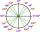What is greater angle? -0.7 radians or -0.7π radians?
4. Neighbor angle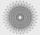For 136° angle calculate size of adjacent angle on one side of a straight line.
5. Angle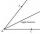Draw angle |∠ ABC| = 130° and built its axis. What angle is between axis angle and arm of angle?
6. Straight lines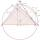Draw two lines c, d so that c || d. On line c mark points A, B, from point A start perpendicular to line c, from point B perpendicular to line c.
7. Compare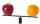Compare with characters >, <, =: 85.57 ? 80.83
8. Triangle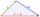Determine if it is possible to construct a triangle with sides 28 31 34 by calculation.
9. Ten fractionsWrite ten fractions between 1/3 and 2/3
10. DoctorsIn the city operates 171 doctors. The city has 128934 citizens. How many citizens are per one doctor?
11. To thousands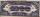Round to thousands following numbers:
12. Roman numerals 2+Add up the number writtens in Roman numerals. Write the results as a roman numbers.
13. ProductResult of the product of the numbers 1, 2, 3, 1, 2, 0 is:
14. Roman numerals +Add up the number writtens in Roman numerals. Write the results as a decimal number.
15. Roman numeralsWrite numbers written in Roman numerals as decimal.
16. Train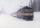The train passes part of the line for 95 minutes at speed 75 km/h. What speed would have to go in order to shorten the driving time of 20 minutes?
17. Addition of Roman numbersAdded together and write as decimal number: LXVII + MLXIV